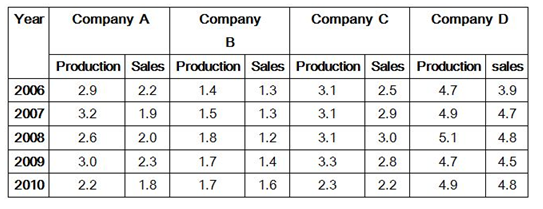# “20-20” Quantitative Aptitude | Crack SBI Clerk 2018 Day-1

Dear Friends, SBI Clerk 2018 Notification has been released we hope you all have started your preparation. Here we have started New Series of Practice Materials specially for SBI Clerk 2018. Aspirants those who are preparing for the exams can use this “20-20” Quantitative Aptitude Questions.

[WpProQuiz 1120]

### Click “Start Quiz” to attend these Questions and view Explanation

Directions(1-5) Study the following series carefully and answer the questions:

1). 7, 4, 5, 12, 52, ?

1. 424
2. 416
3. 442
4. 448
5. None of these
1. 5 7 10 20 35 ?
1. 61
2. 60
3. 66
4. 59
5. 63
1. 4 17 75 210 454 ?
1. 829
2. 847
3. 839
4. 851
5. 833
1. 7 11 25 59 133 ?
1. 286
2. 287
3. 283
4. 295
5. 288
1. 5 7 10 20 35 ?
1. 61
2. 60
3. 66
4. 59
5. 63

Directions (Q.6-10): Study the table and answer the given questions.

Figures regarding number of cars produced and sold (in lakh per year)1. What is the ratio of the total number of cars produced by company C in both the years 2007 and 2010 together, to the total number of cars sold by company A in both the years 2007 and 2009 together?
1. 3 :2
2. 14:11
3. 9 : 7
4. 7 :4
5. 29:18
1. What is the percentage change in the number of unsold cars for company C in the year 2009, compared to the previous year?
1. 23 % increase
2. 500% increase
3. 25% decrease
4. 50% increase
5. 400% increase
1. What is the total number of cars sold by all the given companies together in the year 2008?
1. 10.4 lakh
2. 12.1 lakh
3. 11.8 lakh
4. 11.0 lakh
5. 8.4 lakh
1. What is the average production of company A over all the years?
1. 2.85 lakh
2. 2.90 lakh
3. 2.36 lakh
4. 2.78 lakh
5. 2.58 lakh
1. What is the ratio of the total sales of Company A to that of Company D for the years 2007, 2009 and 2010 taken together?
1. 3:7
2. 4 : 7
3. 10 : 11
4. 7 : 13
5. 14 : 23

Directions (Q. 11-15): what will come in place of question mark (?) in the given questions .

1. ? % of (584.2 – 244.2) = (9)^2+ 21
1. 40
2. 45
3. 30
4. 60
5. 50
1. 58% of 850 + ?= 1224 —603
1. 128
2. 130
3. 138
4. 140
5. None of these
1. 689 x 6156 ÷ 18% of 684 =28 x 250 ÷ 8 + ? + 4300
1. 27295
2. 29275
3. 29527
4. 29725
5. 27925
1. 430% of 650 + (?) of 4.5 – 20% of 2220 = 7292
1. 1298
2. 1098
3. 1089
4. 1198
5. 1398
1. 3/8 of 168 x 15 ÷ 5 + ? = 549 ÷ 9 + 235
1. 189
2. 107
3. 174
4. 296
5. 326
1. Sudip, Pratik and Yash are three cooks working in a restaurant. Yash can prepapre 10 rotis in an hour. The number of rotis prepared by Sudip in 6 hours is the same as the number of rotis prepared by Pratik in 8 hours. All three of them working together can prepare 228 rotis in 6 hours. One day Sudip. Pratik and Yash worked for 5, 6 and 8 hours respectively. What is the total number of rotis prepared by them that day?
1. 236
2. 240
3. 224
4. 232
5. 248
1. A milk man makes a profit of 10% on the sale of milk. If he were to add water such that it makes up to 20% of the mixture, by how many percentage points would his profit increase?
1. 37.5
2. 30
3. 25.5
4. 22
5. 27.5
1. A and B started a business with initial investment of Rs 1300 and Rs 1500 respectively. After 5 months, A added Rs 200. After 4 months, B added Rs 500 and after another 3 months, B added Rs 1000. If at the end of the year. they earned the profit of Rs 35200. Calculate A’s share of profit.
1. 21600
2. 13600
3. 15600
4. 18600
5. 19600
1. Ramesh borrowed Rs 30000 from a bank at a rate of 12% per annum for two years at simple interest and lends the same money to Mahesh at the rate of 12% for two years but he charged compound interest. What is the overall gain by Ramesh?
1. Rs 510
2. Rs 472
3. Rs 432
4. Rs 396
5. Rs 414
1. Mr. Rajbir buys two articles for Rs. 800. He sells first article at a profit of 15% and second article at a loss of 5%. After doing both the transactions Mr. Rajbir realizes that he has neither earned profit nor did he incur any loss. At what cost (in Rs.) did Rajbir sell the loss making article?
1. 550
2. 500
3. 650
4. 600
5. 570